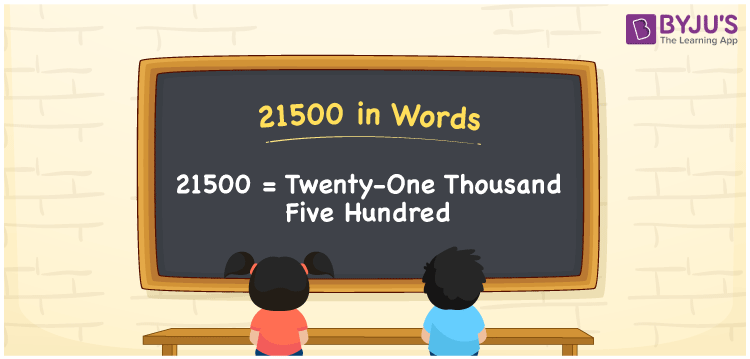# 21500 in Words

We can represent 21500 in words as Twenty-One Thousand Five Hundred. For example, if you saved Rs 21500 in a month, you can write, “I have saved Rupees Twenty-One Thousand Five Hundred in a month”. Number name of any number is written using the ones, tens, hundreds and thousands place of a number. Therefore, a place value chart is important to write 21500 in words. 21500 is a cardinal number because it depicts a specific quantity.

 21500 in words Twenty-One Thousand Five Hundred Twenty-One Thousand Five Hundred in numerical form 21500

## 21500 in English Words

To write numbers in words the most commonly used language is English. Therefore, students must learn how to write number names in English. Hence, the number 21500 in English is written as “Twenty-One Thousand”.## How to Write 21500 in Words?

In order to write the number name of 21500, we have to first determine the place value of each digit. Learn how to write the number name of 21500 in English following the chart given below.

 Ten-Thousands Thousands Hundreds Tens Ones 2 1 5 0 0

Hence, we can write the expanded form as:

2 x Ten-Thousand + 1 x Thousand + 5 x Hundred + 0 × Ten + 0 × One

= 2 × 10000 + 1 × 1000 + 5 x 100 + 0 x 10 + 0 x 1

= 20000 + 1000 + 500 + 0 + 0

= 20000 + 1000 + 500

= Twenty-One Thousand

Therefore, 21500 in words is written as Twenty-One Thousand Five Hundred.

Interesting way of writing 21500 in words

2 = Two

21 = Twenty-One

215 = Two Hundred and Fifteen

2150 = Two Thousand One Hundred Fifty

21500 = Twenty-One Thousand Five Hundred

Thus, the word form of the number 21500 is Twenty-One Thousand Five Hundred.

21500 is a natural number that is the successor of 21499 and the predecessor of 21501

• 21500 in words – Twenty-One Thousand Five Hundred
• Is 21500 an odd number? – No
• Is 21500 an even number? – Yes
• Is 21500 a perfect square number? – No
• Is 21500 a perfect cube number? – No
• Is 21500 a prime number? – No
• Is 21500 a composite number? – Yes

## Frequently Asked Questions on 21500 in Words

Q1

### Write 21500 in English.

21500 in English is written as Twenty-One Thousand Five Hundred.
Q2

### Find the value of 6730 + 14770 and express the answer in words.

6730 + 14770 gives 21500. 21500 in words is expressed as Twenty-One Thousand Five Hundred.
Q3

### Is the number 21500 an odd number?

No, the number 21500 is not an odd number.